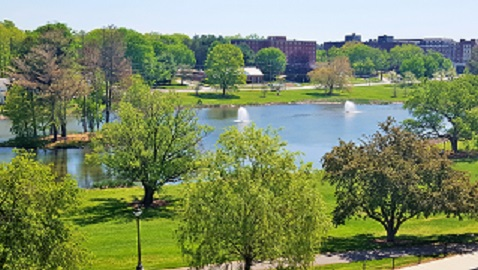Mirror lake, viewed from the Math Department.

I am a Professor in the Department of Mathematics  at the University of Connecticut. Here is my most recent CV.

Research experience:
My research interests are in differential geometry and partial differential equations. A significant part of my recent work has focused on the singularity formation in the Ricci flow. With Jiaping Wang, we studied the asymptotic geometry of four-dimensional Ricci solitons, roughly proving that 4d shrinking Ricci solitons must be either conical or cylindrical at infinity MW1, MW2.

I am currently also interested in understanding how scalar curvature interacts with geometry and analysis on three-dimensional manifolds. Jiaping Wang and I studied volume and spectral comparison estimates for three-dimensional noncompact Riemannian manifolds with scalar curvature bounded below MW3MW4.

Other projects that I worked on concern Green's function estimates on noncompact manifolds MSW1, MSW2, on which I recently collaborated with Chiung-Jue Sung and Jiaping Wang. The three of us recently also studied the area and spectrum of stable minimal hypersurfaces MSW.

Check here for a complete list of my papers on the arXiv.

Teaching experience:
Undergraduate courses: Advanced Calculus I, II, III, IV (Math 2141, Math 2142, Math 2143, Math 2144), Analysis (Math 3150), Differential Equations for Applications (Math 3410), Elements of Topology (Math 3330),  Differential Geometry (Math 3370), Multivariable Calculus (Math 2110), Honors Calculus II (Math 1152), Problem Seminar (Math 3794).

Graduate courses: Introduction to Geometry and Topology I and II (Math 5310 and Math 5311), Topics in Geometry and Topology (Math 5030), Complex Analysis (Math 5120), Topics in Complex Function Theory (Math 5121), Differential Geometry (5360).

Math Problems:  I enjoy solving interesting math problems.
To entertain others like me, I have compiled a list of (hopefully) challenging math problems, many of which are my own work. Enjoy!
Math Problems